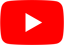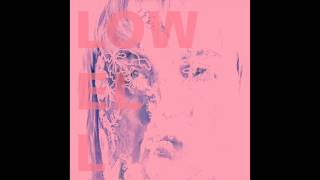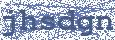The Sun
Lowell

## LOWELL

The Sun Lyrics

Let me be the preacher
Let me be the preacher
Let me be the preacher
Let me be the preacher

I'm gone
I'm gone

Like the sun

I'm gone
I'm gone

Like the sun

I'm gone
I'm gone
Like the sun
Let me be the preacher
Let me be the preacher
Let me be the preacher
Let me be the preacher

I'm gone
I'm gone

Like the sun

I'm gone
I'm gone

Like the sun

I'm gone
I'm gone
Like the sunHottest Lyrics with Videos
a7722ff2b3e1a9c4445deb996df47c1c